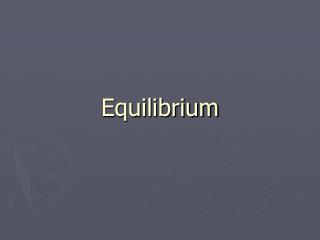DownloadDownload PresentationEquilibrium

# Equilibrium

Télécharger la présentation## Equilibrium

- - - - - - - - - - - - - - - - - - - - - - - - - - - E N D - - - - - - - - - - - - - - - - - - - - - - - - - - -
##### Presentation Transcript

1. Equilibrium

2. Equilibrium Some reactions (theoretically all) are reversible reactions, in which the products take part in a separate reaction to reform the reactants.

3. Equilibrium We show equilibrium in a reaction using an arrow pointing both ways (  ). For example: 2NO2 (g)  N2O4 (g)

4. Equilibrium • Chemical equilibrium is when the rate of the forward reaction is equal to the rate of the reverse reaction.

5. Equilibrium • At equilibrium, the concentrations of reactants and products remain constant over time.

6. Equilibrium The equilibrium constant expresses the relative concentrations of reactants and products at equilibrium. It is Keq.

7. Equilibrium For the reaction aA + bB  cC + dD Keq =[C]c[D]d [A]a[B]b

8. Equilibrium Keq =[C]c[D]d [A]a[B]b • [A],[B],[C] and [D] are molar concentrations • a, b, c and d are the coefficients in the balanced equation

9. Equilibrium Keq =[C]c[D]d [A]a[B]b • When writing equilibrium constant expressions, it is customary to omit units

10. Equilibrium For example: Write the equilibrium expression for this reaction: 2CO(g) + O2 (g)  2CO2(g) Keq =[CO2]2 [CO]2[O2]

11. Equilibrium By plugging in values for concentration, we can determine whether a system is at equilibrium and how it might change as it heads toward equilibrium.

12. Equilibrium Consider this reaction: COCl2 (g)  CO(g) + Cl2(g) Keq = 170 If the concentrations of CO and Cl2 are each 0.15 M and the concentration of COCl2 is 1.1 x 10-3 M, is the reaction at equilibrium? If not, in which direction will it proceed?

13. Equilibrium Set up the equilibrium expression and plug in values: Keq =[CO][Cl2]=(0.15) (0.15) [COCl2] (1.1 x 10-3)

14. Equilibrium Q = 20, which is not the same as 170, so the system is not at equilibrium. To be at equilibrium, we would like to have it get bigger.

15. Equilibrium If Q is to get bigger, we would need more CO and Cl2, so the reaction needs to shift to the right.